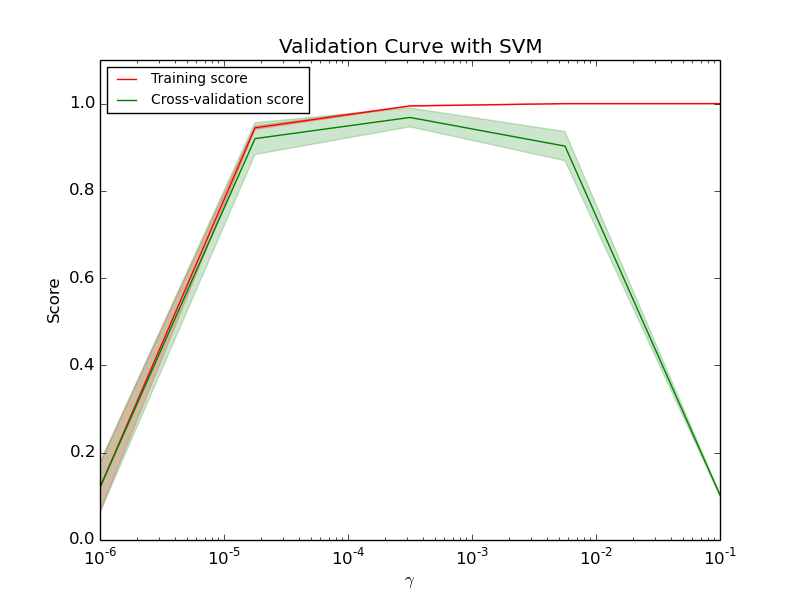This documentation is for scikit-learn version 0.16.1Other versions

If you use the software, please consider citing scikit-learn.

# Plotting Validation Curves¶

In this plot you can see the training scores and validation scores of an SVM for different values of the kernel parameter gamma. For very low values of gamma, you can see that both the training score and the validation score are low. This is called underfitting. Medium values of gamma will result in high values for both scores, i.e. the classifier is performing fairly well. If gamma is too high, the classifier will overfit, which means that the training score is good but the validation score is poor.Python source code: plot_validation_curve.py

print(__doc__)

import matplotlib.pyplot as plt
import numpy as np
from sklearn.datasets import load_digits
from sklearn.svm import SVC
from sklearn.learning_curve import validation_curve

X, y = digits.data, digits.target

param_range = np.logspace(-6, -1, 5)
train_scores, test_scores = validation_curve(
SVC(), X, y, param_name="gamma", param_range=param_range,
cv=10, scoring="accuracy", n_jobs=1)
train_scores_mean = np.mean(train_scores, axis=1)
train_scores_std = np.std(train_scores, axis=1)
test_scores_mean = np.mean(test_scores, axis=1)
test_scores_std = np.std(test_scores, axis=1)

plt.title("Validation Curve with SVM")
plt.xlabel("$\gamma$")
plt.ylabel("Score")
plt.ylim(0.0, 1.1)
plt.semilogx(param_range, train_scores_mean, label="Training score", color="r")
plt.fill_between(param_range, train_scores_mean - train_scores_std,
train_scores_mean + train_scores_std, alpha=0.2, color="r")
plt.semilogx(param_range, test_scores_mean, label="Cross-validation score",
color="g")
plt.fill_between(param_range, test_scores_mean - test_scores_std,
test_scores_mean + test_scores_std, alpha=0.2, color="g")
plt.legend(loc="best")
plt.show()


Total running time of the example: 39.23 seconds ( 0 minutes 39.23 seconds)